Place Value: Ones & Tens Math Video for Kids - K, 1st, & 2nd Grades
1%
It was processed successfully!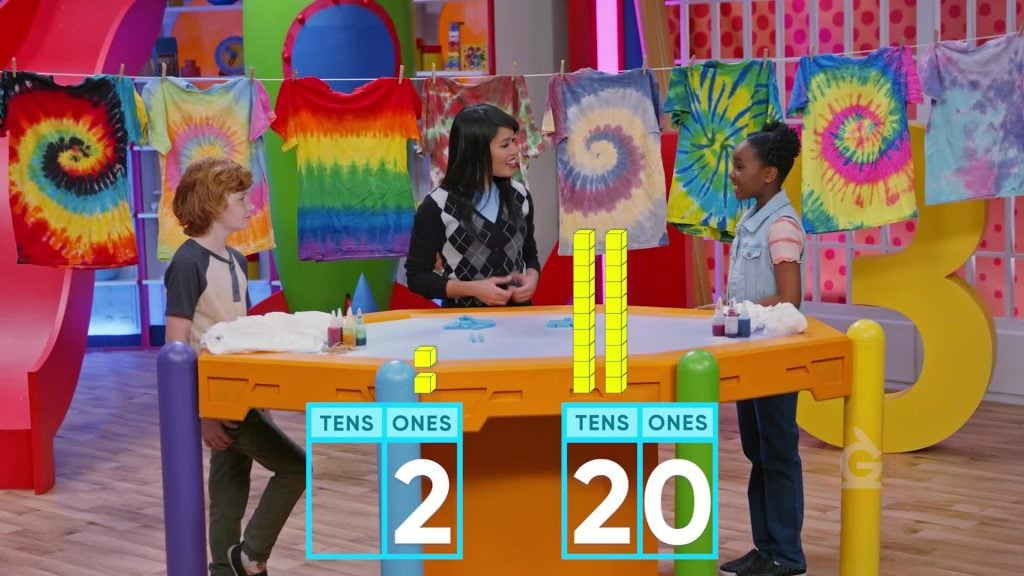WHAT IS PLACE VALUE?

Numbers have one or more digits. The digits in 43 are 4 and 3. Place value is the value of a digit based on its place in the number.

To better understand place value…

WHAT IS PLACE VALUE?. Numbers have one or more digits. The digits in 43 are 4 and 3. Place value is the value of a digit based on its place in the number. To better understand place value…

## LET’S BREAK IT DOWN!

### Identify the tens and ones places in numbers.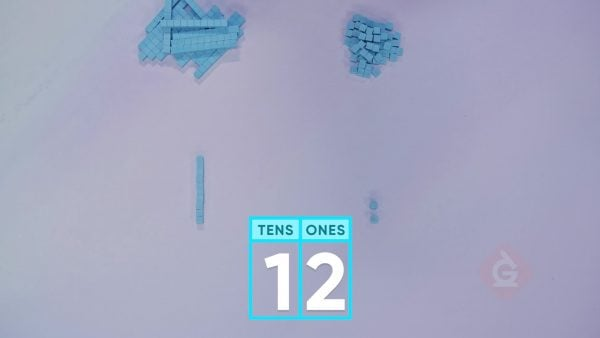The 1 in the number 12 is in the tens place. The 2 in 12 is in the ones place. 95 has a 9 in the tens place and a 5 in the ones place.

Identify the tens and ones places in numbers. The 1 in the number 12 is in the tens place. The 2 in 12 is in the ones place. 95 has a 9 in the tens place and a 5 in the ones place.

### You can break numbers into tens and ones.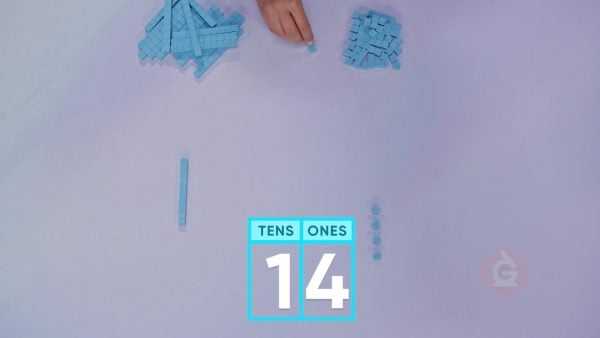The number 14 has a 1 in the tens place. It has a 4 in the ones place. There are 1 ten and 4 ones in 14. 27 is 2 tens and 7 ones.

You can break numbers into tens and ones. The number 14 has a 1 in the tens place. It has a 4 in the ones place. There are 1 ten and 4 ones in 14. 27 is 2 tens and 7 ones.

### There are zeroes in numbers.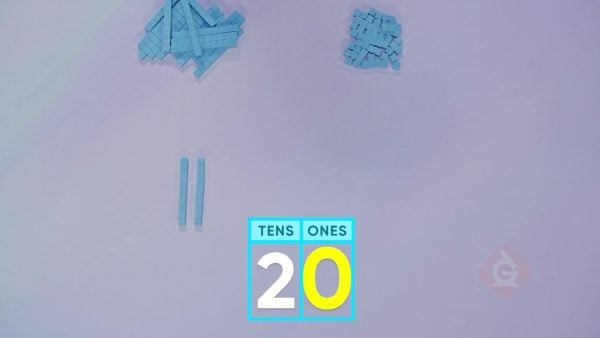0 is an important number. The zero in 20 tells us that there are no ones. That helps us know that the 2 in 20 is in the tens place. 20 is 2 tens and 0 ones.

There are zeroes in numbers. 0 is an important number. The zero in 20 tells us that there are no ones. That helps us know that the 2 in 20 is in the tens place. 20 is 2 tens and 0 ones.

### Compare 46 and 64.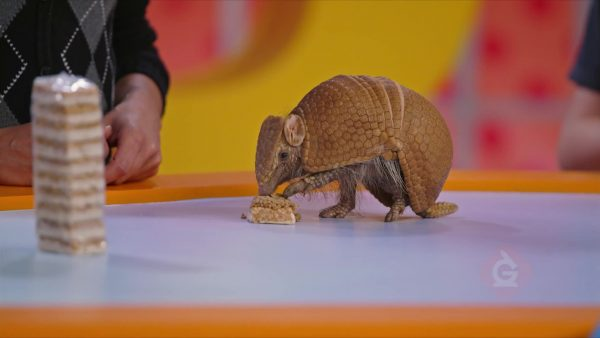46 has 4 tens and 6 ones. 64 has 6 tens and 4 ones. 46 and 64 have the same digits but have very different values. The place of a digit shows its value.

Compare 46 and 64. 46 has 4 tens and 6 ones. 64 has 6 tens and 4 ones. 46 and 64 have the same digits but have very different values. The place of a digit shows its value.

### 79 and 1 More.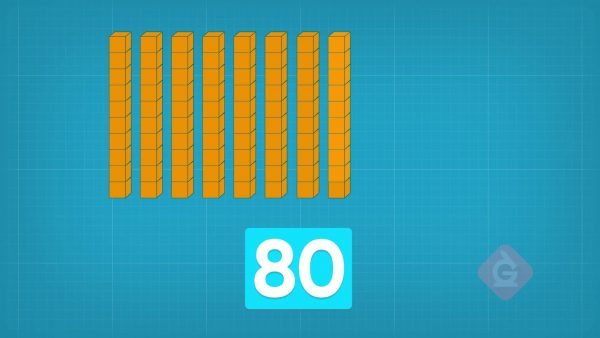Suppose you have 79 crackers. That’s 7 tens and 9 ones. You add another cracker. Now there are 7 tens and 10 ones. 10 ones is the same as 1 ten. Then you have 8 tens. 8 tens is 80. There are 80 crackers.

79 and 1 More. Suppose you have 79 crackers. That’s 7 tens and 9 ones. You add another cracker. Now there are 7 tens and 10 ones. 10 ones is the same as 1 ten. Then you have 8 tens. 8 tens is 80. There are 80 crackers.

## PLACE VALUE: ONES & TENS VOCABULARY

Place Value
The value of a digit based on its position in a number.
Base-ten blocks
Blocks that can be used to represent the number of tens and ones in a number.
Ones
A place in the place-value system that has a value of 1. In the number 23, 3 is in the ones place.
Tens
A place in the place-value system that has a value of 10. In the number 23, 2 is in the tens place.
Digit
Numerals from 0 to 9 that form part of a number.
Groups
Objects that have the same attribute are put together to form a group. For example, in the place-value system, 7 ones can be put together in a group of ones.

## PLACE VALUE: ONES & TENS DISCUSSION QUESTIONS

### [Show students 5 tens blocks.] Write the number that the blocks represent. How do you know?

The blocks show 5 tens. 5 tens is 50. The number 50 has a 5 in the tens place and a 0 in the ones place.

### [Show students 3 tens blocks and 4 ones blocks.] Write the number that the blocks represent. How do you know?

The blocks show 3 tens and 4 ones. The number 34 has a 3 in the tens place and 4 in the ones place.

### How can you represent 63 with the base-ten blocks?

63 has 6 tens and 3 ones. I can show 63 with 6 tens blocks and 3 ones blocks.

### What is the value of 4 in 47? How do you know?

4 tens or 40; 4 is in the tens place, so its value is 4 tens or 40.

### What number do you get when you add 1 to 29? Explain.

29 is 2 tens and 9 ones. 1 more one makes 2 tens and 10 ones. 10 ones is the same as 1 more ten. That gives me 3 tens. 30 is 3 tens. So the answer is 30.
X

## Success

We’ve sent you an email with instructions how to reset your password.
Ok